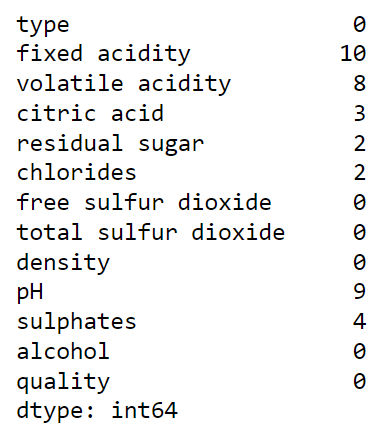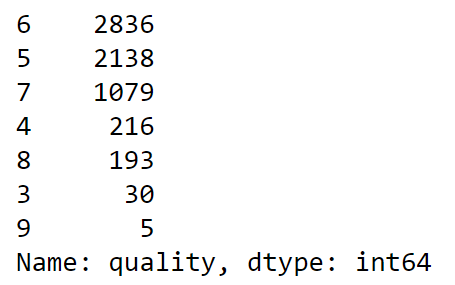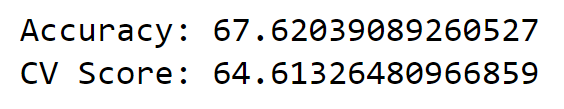top of page
• Hackers Realm

# Wine Quality Prediction Analysis using Python | Classification | Machine Learning Project Tutorial

Updated: Jun 5

Embark on a thrilling journey of wine quality prediction analysis using Python. This captivating blog tutorial explores classification techniques and machine learning algorithms. Uncover the secrets of data preprocessing, feature engineering, and model evaluation. Dive into the world of classification algorithms like logistic regression, decision trees, and random forests to predict wine quality. Enhance your Python programming, data analysis, and machine learning skills through this comprehensive tutorial. Join us as we uncork the wonders of wine quality prediction! #WineQualityPrediction #Python #Classification #MachineLearning #DataAnalysisWine Quality Prediction

In this project tutorial, we can create both classification and regression models for this project using python. You can select the model of your choice based on the evaluation metrics that the contest proposes. In the case of an accuracy metrics, you can create a classification model. And in the case of an error metrics, you can create a regression model.

You can watch the video-based tutorial with step by step explanation down below.

## Dataset Information

The two datasets are related to red and white variants of the Portuguese "Vinho Verde" wine. These datasets can be viewed as classification or regression tasks. The classes are ordered and not balanced (e.g. there are many more normal wines than excellent or poor ones). Outlier detection algorithms could be used to detect the few excellent or poor wines. Also, we are not sure if all input variables are relevant. So it could be interesting to test feature selection methods. Two datasets were combined and a few values were randomly removed.

### Attribute Information:

Input variables (based on physicochemical tests): 1 - fixed acidity 2 - volatile acidity 3 - citric acid 4 - residual sugar 5 - chlorides 6 - free sulfur dioxide 7 - total sulfur dioxide 8 - density 9 - pH 10 - sulphates 11 - alcohol Output variable (based on sensory data): 12 - quality (score between 0 and 10)

## Import modules

First, we have to import all the basic modules we will be needing for this project.

```import pandas as pd
import numpy as np
import seaborn as sns
import matplotlib.pyplot as plt
import warnings
%matplotlib inline
warnings.filterwarnings('ignore')```
• pandas - used to perform data manipulation and analysis

• numpy - used to perform a wide variety of mathematical operations on arrays

• matplotlib - used for data visualization and graphical plotting

• seaborn - built on top of matplotlib with similar functionalities

• %matplotlib - to enable the inline plotting

• warnings - to manipulate warnings details

• filterwarnings('ignore') is to ignore the warnings thrown by the modules (gives clean results)

```df = pd.read_csv('winequality.csv')Wine Quality Dataset
• The Input attributes are in numerical forms.

• We have to predict the output variable "quality".

```# statistical info
df.describe()```Statistical Information of Dataset
• We will fill the missing values using the mean values.

```# datatype info
df.info()```Datatype Information
• Only one input attribute is an object and the others are in float.

• Output attribute is integer datatype. We can read the attribute as a classifier or regressor because it is in a particular range.

## Preprocessing the dataset

Let us check for NULL values in the dataset.

```# check for null values
df.isnull().sum()```Count of Null Values
• We observe seven attributes with missing values.

Let us fill the missing values.

```# fill the missing values
for col, value in df.items():
if col != 'type':
df[col] = df[col].fillna(df[col].mean())```
`df.isnull().sum()`• Since attribute 'type' is an object datatype. We have to ignore it using the if condition.

• We use mean() to fill the mean values of that particular attribute.

• To fill more missing values, you can also use advanced filling techniques (For example deriving values using features of other attributes).

## Exploratory Data Analysis

Let us explore the boxplot of the attributes, to check the outliers.

```# create box plots
fig, ax = plt.subplots(ncols=6, nrows=2, figsize=(20,10))
index = 0
ax = ax.flatten()

for col, value in df.items():
if col != 'type':
sns.boxplot(y=col, data=df, ax=ax[index])
index += 1Box Plot for Attributes
• We observe outliers from a few attributes.

• Eliminating these outliers will improve the accuracy of the model.

• Since it won't affect the outcome of the project, we will ignore this outlier.

Let us explore the distribution plot of all numerical attributes.

```# create dist plot
fig, ax = plt.subplots(ncols=6, nrows=2, figsize=(20,10))
index = 0
ax = ax.flatten()

for col, value in df.items():
if col != 'type':
sns.distplot(value, ax=ax[index])
index += 1Distribution Plot for Attributes
• We observe graphs of good range. However, we can improve a few attributes by removing outliers from that particular attributes.

• The column 'Free sulfur dioxide' is slightly right-skewed. Thus we need to normalize it using log transformation.

Log transformation

Log transformation helps to make the highly skewed distribution to less skewed.

```# log transformation
df['free sulfur dioxide'] = np.log(1 + df['free sulfur dioxide'])```
`sns.distplot(df['free sulfur dioxide'])`• We can observe a Normal distribution in a form of a bell curve.

Let us explore the datasets count in different wines.

`sns.countplot(df['type'])`Distribution of Wine Type
• Most datasets belong to the white wines category.

`sns.countplot(df['quality'])`Distribution of Wine Quality
• Although the quality ranges from 0 to 10. However, for this dataset, it is in the range of 3 to 9.

• The middle classes have higher counts. Therefore the entire model will be biased toward these three classes.

• Since the data are imbalanced through the classes, we may need to perform class-balancing after splitting the data.

## Correlation Matrix

A correlation matrix is a table showing correlation coefficients between variables. Each cell in the table shows the correlation between two variables. The value is in the range of -1 to 1. If two variables have a high correlation, we can neglect one variable from those two.

```corr = df.corr()
plt.figure(figsize=(20,10))
sns.heatmap(corr, annot=True, cmap='coolwarm')```Correlation Matrix
• The output attribute 'quality' shows a positive correlation with 'alcohol'.

• Additionally, we observe a positive correlation between 'free sulfur dioxide' and 'total sulfur dioxide'.

• You can drop the attribute 'density' and 'free sulfur dioxide' to remove some features.

## Input Split

Let us split the dataset before balancing the class.

```X = df.drop(columns=['type', 'quality'])
y = df['quality']```
• Storing the input attributes in X variable and storing the output attribute in y variable

## Class Imbalancement

We use smote to balance the class ratio.

`y.value_counts()`• It shows the count of data values for each class.

• The oversample function generates new features from minority classes.

```from imblearn.over_sampling import SMOTE
oversample = SMOTE(k_neighbors=4)
# transform the dataset
X, y = oversample.fit_resample(X, y)```
`y.value_counts()`• Now all the classes have oversampled to the upper value.

• Further, you can get a uniform dataset.

• To use this dataset for multi-classification, you can specify percentages in a dictionary. Afterwards, you can get that specific percentage data for each class.

• Additionally, you can combine the oversample function with the random undersample function to get a good data.

## Model Training

Let us perform the model training and testing.

• You can use classification or regressor to train the model.

• Here, we will use classification to train our model.

```# classify function
from sklearn.model_selection import cross_val_score, train_test_split
def classify(model, X, y):
x_train, x_test, y_train, y_test = train_test_split(X, y, test_size=0.25, random_state=42)
# train the model
model.fit(x_train, y_train)
print("Accuracy:", model.score(x_test, y_test) * 100)

# cross-validation
score = cross_val_score(model, X, y, cv=5)
print("CV Score:", np.mean(score)*100)```
• X contains input attributes and y contains the output attribute.

• We use cross val score() for better validation of the model.

• np.mean() will give the average value of 5 scores.

• Let's train our data with different models.

Logistic Regression.

```from sklearn.linear_model import LogisticRegression
model = LogisticRegression()
classify(model, X, y)```• Here, logistic regression is a classification model

Decision Tree

```from sklearn.tree import DecisionTreeClassifier
model = DecisionTreeClassifier()
classify(model, X, y)```• The result has improved.

Random Forest.

```from sklearn.ensemble import RandomForestClassifier
model = RandomForestClassifier()
classify(model, X, y)```• Random forest shows better results than the decision tree classifier.

Extra Trees.

```from sklearn.ensemble import ExtraTreesClassifier
model = ExtraTreesClassifier()
classify(model, X, y)```• Both the accuracy and cv are better than the random forest classifier.

XGBoost.

```import xgboost as xgb
model = xgb.XGBClassifier()
classify(model, X, y)```LightGBM.

```import lightgbm
model = lightgbm.LGBMClassifier()
classify(model, X, y)```• Both accuracy and cv score is less than the Extra trees classifier.

## Final Thoughts

• Out of all the classifiers, Extra tress shows better results for the dataset.

• Without balancing the data, the advanced model displays poor results.

• You can remove outliers and drop correlated attributes to improve model performance.

• Additionally, you can use oversampling combined with random undersampling and try to normalize the dataset.

In this article, we analyzed the dataset for wine quality using machine learning. Likewise, we discussed the methods to balance the class. We have used the feature selection method to analyze the dataset.

Get the project notebook from here